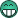# Lie groups and Lie algebras: A local logarithm

Discussion générale entre passionnés et amateurs de mathématiques sur des sujets mathématiques variésortollj
Membre Rationnel
Messages: 536
Enregistré le: 13 Mai 2009, 10:28

### Lie groups and Lie algebras: A local logarithm

Bonjour
je voulais ajouter le commentaire ci dessous (partie en Anglais), mais pour une raison que j'ignore ,
je ne peux mettre un commentaire.
si j'ajoute un commentaire celui ci apparait mais disparait si je rafraichi la page de la video.
si quelqu'un ici a la patience de lire la Video et de repondre a ma question ci dessous .

Lie groups and Lie algebras: A local logarithm

I allow myself to add here a practical example in dimension 2 to illustrate this video,
maybe it can help other people like me who had difficulty understanding this video.

https://sagecell.sagemath.org/?q=fqhjtp

but I don't understand the last part of this video. I understand that d A_ {i, j) / d A_ {i, j) = 1
but I don't understand why d A_ {i, j) / d A_ {i, k) = 0.
imagine that A = matrix ([[e ^ (x0 - x1) -e ^ (x0 - x1)], [e ^ x0 e ^ x1]])
and take d A_ {0,0) / d A_ {1,0) => d {e ^ (x0 - x1)} / d {e ^ x0}, then if e ^ x0 varies then e ^ (x0 - x1) also varies
and therefore d A_ {0,0) / d A_ {1,0)! = 0 ? but maybe this does not apply to any matrices A which are commuting ?
what particular type of matrix A does d A_ {i, j) / d A_ {n, m) = 0 apply to?

but i guess there is something important here that i didn't understand ! Sorry if my question is stupid.

Lie group and Lie Algebra playList
si j'avais su j'aurais pas venu.mathelot
Habitué(e)
Messages: 12730
Enregistré le: 08 Juin 2006, 09:55

### Re: Lie groups and Lie algebras: A local logarithm

ortollj a écrit: I understand that d A_ {i, j) / d A_ {i, j) = 1

bonjour,
il y a une erreur LaTeX {i,j) commence par une accolade et termine par une parenthèseortollj
Membre Rationnel
Messages: 536
Enregistré le: 13 Mai 2009, 10:28

### Re: Lie groups and Lie algebras: A local logarithm

mathelot a écrit:
ortollj a écrit: I understand that d A_ {i, j) / d A_ {i, j) = 1

bonjour,
il y a une erreur LaTeX {i,j) commence par une accolade et termine par une parenthèse

oui effectivement ,bon je pense que malgré cela on comprends le sens de ma question ?
[edited 20h27 ] :enfin si celle ci a du sens !Ah oui merci c'est peut etre pour cela que mon commentaire est a été rejeté.
[edited 20h22]
Non apres reflexion je ne crois pas car les commentaires youtube ne prennent pas en compte le Latex
si j'avais su j'aurais pas venu.

Retourner vers ⚜ Salon Mathématique

### Qui est en ligne

Utilisateurs parcourant ce forum : Aucun utilisateur enregistré et 8 invités

Tu pars déja ?

Fais toi aider gratuitement sur Maths-forum !

Créé un compte en 1 minute et pose ta question dans le forum ;-)

Identification

Pas encore inscrit ?

Ou identifiez-vous :